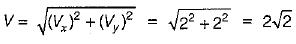Courses

# Fluid Flow Kinematics - 1

## 10 Questions MCQ Test Mock Test Series for Civil Engineering (CE) GATE 2020 | Fluid Flow Kinematics - 1

Description
This mock test of Fluid Flow Kinematics - 1 for Civil Engineering (CE) helps you for every Civil Engineering (CE) entrance exam. This contains 10 Multiple Choice Questions for Civil Engineering (CE) Fluid Flow Kinematics - 1 (mcq) to study with solutions a complete question bank. The solved questions answers in this Fluid Flow Kinematics - 1 quiz give you a good mix of easy questions and tough questions. Civil Engineering (CE) students definitely take this Fluid Flow Kinematics - 1 exercise for a better result in the exam. You can find other Fluid Flow Kinematics - 1 extra questions, long questions & short questions for Civil Engineering (CE) on EduRev as well by searching above.
QUESTION: 1

### Local acceleration in fluid-flow situations exists only when

Solution: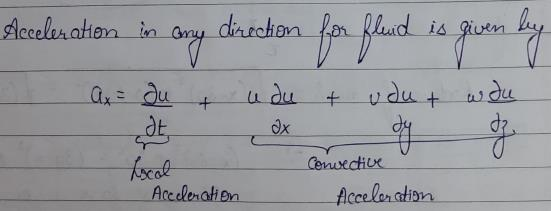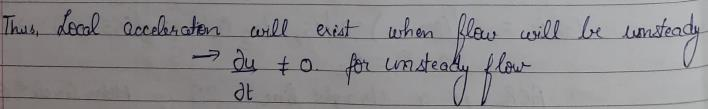QUESTION: 2

Solution:
QUESTION: 3

### A free vortex

Solution:
QUESTION: 4

Stream lines, streak lines and path lines are all identical in case of

Solution:
QUESTION: 5

In irrotational flow of an ideal fluid

Solution:
QUESTION: 6

Match List-I (Format of representation) with List-ll (Context/Relevant to) and select the correct answer using the codes given below the lists: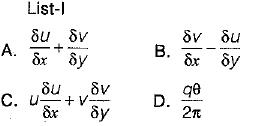List-ll
1. Relevant to a velocity potential
2. Rate of rotation about a relevant axis
3. Pressure gradient in a relevant direction
4. Continuity of flow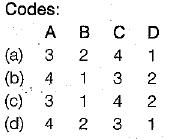Solution:
QUESTION: 7

A two-dimensional flow is described by velocity components u = 2x and v = - 2y. The discharge between points (1,1) and (2, 2) is equal to

Solution: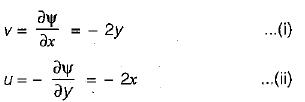Integrating (i), we get,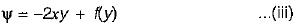Differentiating (iii) w.r.t. y, we get,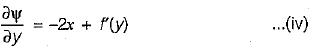Equating (ii) and (iv), we get,
f'(y) = 0    ....... (v)
Integrating (v), we get,
f(y) = C
where C is a numerical constant which can be treated as zero.
From (iii),. we get,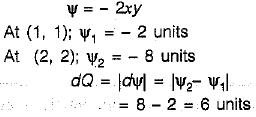QUESTION: 8

Consider the following statements in respect of two-dimensional incompressible flow with velocity components u and v in x and y directions respectively:
1. The continuity equation is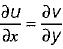2. The acceleration in x-direction is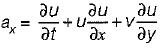3. The condition of irrotationality is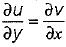4. The equation of a streamline is udy = -vdx

Which of these statements are correct?

Solution:

For two dimensional incompressible flow, :
(i) the continuity equation is'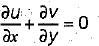(ii) the equation of a stream line is vdx - udy = 0

QUESTION: 9

An imaginary tangent at a point which shows the direction of velocity of a liquid particle at that point is

Solution:

A path-line may be defined as the line traced by a single fluid particle as it moves over a period of time, Thus a path-line will show the direction of velocity of the same fluid particle at successive instants of time. As indicated earlier a streamline on the other hand shows the direction of velocity of a number of fluid particles at the same instant of time. A fluid particle always moves tangentially to the streamline, and in the case of steady flow since there is no change in direction of the velocity vector at any point with time, the streamline is fixed in space. Therefore in steady flow the pathlines and streamlines are identical. However, in unsteady flow since the direction of velocity vector at any point may change with time, streamline may shift in space from instant to instant. A particle then follows one streamline at one instant and another at the next instant and so on, so that the path of the particle may have no resemblance to any given instantaneous streamline. In other words, in unsteady flow path-lines and streamlines are different.

QUESTION: 10

A two dimensional flow field is given by stream function ψ = x2 - y2. The magnitude of absolute velocity at a point (1, 1) is

Solution: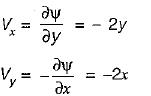at (1, 1) Vx = -2 m/s = Vy